# Ensemble Learning - Bagging and Random Forest¶

Oct. 19, 2018 $\newcommand{\bs}{\boldsymbol}$ $\newcommand{\argmin}{\underset{\bs{#1}}{\text{arg min}}\,}$ $\newcommand{\argmax}{\underset{\bs{#1}}{\text{arg max}}\,}$ $\newcommand{\tr}{^{\top}}$ $\newcommand{\norm}{\left|\left|\,#1\,\right|\right|}$ $\newcommand{\given}{\,|\,}$

Previously, I talked about trees, AdaBoost, and gradient boosting. To complete the series, I will talk about two remaining popular methods called bagging and random forest. Boosting, bagging, and random forest are examples of ensemble learning, where the decision is made not from one, but a collection of - sometimes weak - classifiers. Personally, I am a huge fan of ensemble learning because it uses the similar idea as in the Central Limit Theorem (CLT), which relies on the fact that the variance (i.e. uncertainty) of the sample mean will decrease at the rate proportional to $\sqrt{n}$ where $n$ is the sample size. This is analogous to having a committee, where each committee member is a classifier. In most cases, decisions made from a large committee of experts are usually more reliable and consistant than those made from a single person.

To be consistant to the previous posts, we will focus on classification tree first. Suppose that for a classification problem the response variable $Y$ takes on values $1,2,...,K$. The basic idea behind fitting a classification tree is to follow a top-down, greedy approach that successively splits the feature space, where each binary split of a terminal node is made to minimizes the weighted Gini impurity measure:

$$w_1G_1 + w_2 G_2,$$

where $w_1$ and $w_2$ are weights associated to two resulting rectangles from a binary split and $G_m$ is the Gini index defined by

$$G_m = \sum_{k=1}^K \hat{p}_{mk}(1-\hat{p}_{mk}),$$

where $\hat{p}_{mk}$ is the proportion of class $k$ in the terminal rectangle $R_m$.

## Bagging¶

There is a plethora of advantanges for using trees. For example, the binary decision-making process has more resemblance to human decision-making. Also, there is not many "formulas" involved, making it more intepretable to non-experts than, say, linear regression. Unfortunately, a single tree usually result in a low flexibility in fitting the data. Also overfitting is an issue if the tree is split too many times.

If we have a lot of training sets, by the CLT, the variance problem can be fixed by averaging a set of predictions made by the tree, but we generally cannot have access to multiple training sets.

Instead, we can use bootstrap, by repeatedly sampling from the training data with replacement. Suppose we generate $N$ bootstrapped training sets. We then fit a classification tree on the $i$th boostrapped training set to get a prediction $\hat{f}_{i}(\bs{x})$. Then we form a set containing all $N$ predictions from all bootstrapped samples. The final prediction is made by taking the majority vote, i.e.,

$$\widehat{f}_{\text{bag}}(\bs{x}) = \argmax{k} \sum_{i=1}^N \left[I\left(\hat{f}_{i}\left(\bs{x}\right)= k\right)\right]\tag{1}$$

For regression problem, the final prediction is simply the average of all predicted values.

Another benefit of bagging is that the test error can be estimated right out of the bag - pun intended. The reason for that on average, each bootstrapped sample will use around 63% of the observations. The most straightforward way to see that is by Monte Carlo simulation. We implement it as follows.

In :
import numpy as np

# Draw N random numbers from 1,...,N with replacement
def draw(N):
boot = np.random.randint(1, N+1, N)
return len(np.unique(boot))/N

# Draw N random numbers 10000 times
def Monte_Carlo(N, num_trials=10000):
proportions = []

for i in range(num_trials):
proportions.append(draw(N))

mean = np.mean(proportions)
std = np.std(proportions)

print('N:', N)
print('Mean:', mean)
print('Confidence Interval: ({}, {})'.format(mean-1.96*std, mean+1.96*std))
print('--------')

In :
# Run simulation for different sample size
for N in [5, 10, 100, 1000, 10000, 100000]:
Monte_Carlo(N)

N: 5
Mean: 0.66938
Confidence Interval: (0.3894468769706593, 0.9493131230293407)
--------
N: 10
Mean: 0.6511899999999998
Confidence Interval: (0.455086305210126, 0.8472936947898737)
--------
N: 100
Mean: 0.6335280000000001
Confidence Interval: (0.5724497575679719, 0.6946062424320283)
--------
N: 1000
Mean: 0.6323075
Confidence Interval: (0.6130252092919436, 0.6515897907080564)
--------
N: 10000
Mean: 0.6321464299999999
Confidence Interval: (0.6259728110435051, 0.6383200489564947)
--------
N: 100000
Mean: 0.6321254230000001
Confidence Interval: (0.6301649792855756, 0.6340858667144246)
--------


Based on the results, as long as we have more than 100 data points, there will most likely be around $100\%-63\% =27\%$ of the original data points untouched by the bootstrap sample. But as I was writing the simulation, one thing struck me:

It looks like the expected value is getting close to $1-e^{-1} \approx 0.63212$. Incredible!

This suggests that a closed-form solution exists. To find it, let $N$ denote the total number of samples in the dataset. Also, denote by the random variable $X$ the proportion of distinct elements drawn. Define the Bernoulli random variables $Y_i$ such that

$$Y_i = \begin{cases} 1 & \text{ if the ith observation is drawn } \\ 0 & \text{ otherwise.} \quad \text{ for } i=1,...,N \end{cases}$$

We can now cleverly express $X$ as a combination of $Y_i$'s, and using the linearity property of the expected value, we have

$$X = \frac{1}{N}\sum_{i=1}^N Y_i \implies E(X) = E(Y_i).$$

For each $i$, we can compute the expected value of $Y_i$ as

\begin{aligned} E(Y_i) &= P(Y_i=1) \\ &= 1 - P(Y_i=0) \\ &= 1 - P(\text{ith observation is not drawn}) \\ &= 1 - \left(\frac{N-1}{N}\right)^N \\ &= 1- \left( 1- \frac{1}{N}\right)^N \overset{N\to \infty}{\to} 1-e^{-1} \end{aligned}

So for each bagged tree $\hat{f}_{i}$, we let $O_i$ be the set of the roughly 27% of data that are not used. The set $O_i$ is also referred to the out-of-bag (OOB) samples for the $i$th tree. Now for training examples $\bs{x}^{(1)}, \bs{x}^{(2)}, ..., \bs{x}^{(N)}$, we can predict each one using the trees such that the sample $\bs{x}^{(i)}$ was in $O_i$. That is

$$\hat{f}_{\text{CV}}(\bs{x}^{(i)}) = \argmax{k} \sum_{\{i\,:\,\bs{x}^{(i)}\in O_i\}} \left[I\left(\hat{f}_{i}\left(\bs{x}\right)= k\right)\right]$$

Given training labels $y^{(1)},y^{(2)},..., y^{(N)}$, we can conveniently compute the misclassification rate as an estimate for the test error:

$$L = \frac{1}{N}\sum_{i=1}^N I\left(y^{(i)} = \hat{f}_{\text{CV}}(\bs{x}^{(i)})\right).$$

If we go a step back and find the probabilities that each training example belongs to any of the $K$ classes, we can use the multinomial cross entropy as the error measure.

## Implementation¶

We will be testing on the Iris dataset. The following is data preparation.

In :
import numpy as np
import matplotlib.pyplot as plt

data = iris['data']
Y = iris['target']

print('Training data has dimension', data.shape)
print('Training label has dimension', Y.shape)

Training data has dimension (150, 4)
Training label has dimension (150,)


For demonstration purpose, let's use principal component analysis to reduce the dimension of the dataset to 2.

In :
from sklearn.preprocessing import StandardScaler
from sklearn.decomposition import PCA

# Function that standardizes the data
def standardize(data):
scaler = StandardScaler()
scaler.fit(data)
scaled_data = scaler.transform(data)
return scaled_data

# Define PCA transform function
def pca_transform(data, dimension=2):
scaled_data = standardize(data)
pca = PCA(n_components = dimension)
pca.fit(scaled_data)
x_pca = pca.transform(scaled_data)
return x_pca, pca.explained_variance_ratio_

In :
# PCA plot
X, explained_var = pca_transform(data)
print('Explained variance from Principal Component 1:', explained_var)
print('Explained variance from Principal Component 2:', explained_var)
plt.scatter(X[:,0], X[:,1], c=Y, cmap=plt.cm.Spectral, edgecolors='k')
plt.show()

Explained variance from Principal Component 1: 0.7277045209380135
Explained variance from Principal Component 2: 0.2303052326768062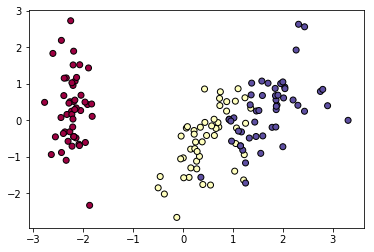Doing a PCA here is a good idea since the first two principal components captures about 95% of the total variance. Plus we can visualize the dataset in 2D! Let's define a function that plots the decision boundary.

In :
# Define a function that plots decision boundary of 2D classification problem
def plot_decision_boundary(model, data, label):

#plt.figure(figsize=[10,10])
x_min, x_max = data[:, 0].min()-0.1, data[:, 0].max()+0.1
y_min, y_max = data[:, 1].min()-0.2, data[:, 1].max()+0.2
xx, yy = np.meshgrid(np.arange(x_min, x_max, 0.02), np.arange(y_min, y_max, 0.02))
Z = model.predict(np.c_[xx.ravel(), yy.ravel()])

# Use a contour plot to show the decision boundary
Z = Z.reshape(xx.shape)
plt.contourf(xx, yy, Z, cmap=plt.cm.Spectral)

# Plot also the training points
plt.scatter(data[:, 0], data[:, 1], c=label, cmap=plt.cm.Spectral, edgecolors='k')
plt.title('Decision boundary')


We are ready to fit a bagging classifier. Here we will fit 100 bootstrapped trees and compute the OOB score discussed earlier.

In :
from sklearn.ensemble import BaggingClassifier

bag = BaggingClassifier(oob_score=True, n_estimators=100)
bag.fit(X, Y)
print('OOB score is', bag.oob_score_)
plot_decision_boundary(bag, X, Y)

OOB score is 0.9133333333333333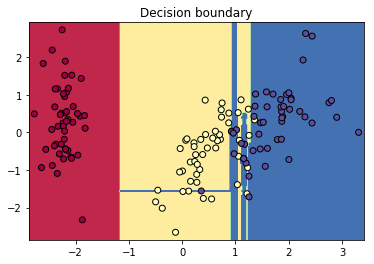For comparison, let's look at the decision boundary from Support Vector Machine, which is clearly a better choice for this dataset than bagged trees.

In :
from sklearn.svm import SVC

svc = SVC()
svc.fit(X, Y)
plot_decision_boundary(svc, X, Y)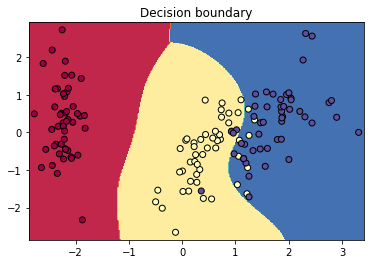Let's check logistic regression.

In :
from sklearn.linear_model import LogisticRegression

glm = LogisticRegression()
glm.fit(X, Y)
plot_decision_boundary(glm, X, Y)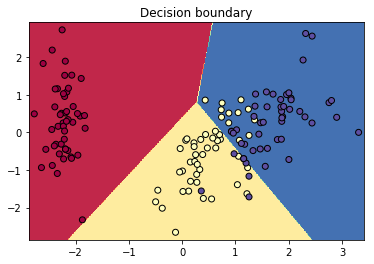## Random Forest¶

One problem about using decision trees is overfitting. As seen from the decision boundary, bagging classifier demonstrates slight overfitting, even though Iris is a very well behaved dataset. Random forest is an upgrade to bagged trees by decorrelating the trees. We know from the Central Limit Theorem that by adding uncorrelated random variables, the variance goes down!

The idea behind random forest is as follows.

• Similar to bagging, we build decision trees on bootstrapped samples of the training data.
• When building each decision tree, each time a binary split is used, a random subset of $m$ features is selected (from $p$ total features).
• A new set of $m$ features is selected for each binary split. (Usually $m \approx \sqrt{p}$.)

Let's do a GridSearch before fitting the model.

In :
from sklearn.ensemble import RandomForestClassifier
from sklearn.model_selection import GridSearchCV

param_grid = {'n_estimators': [10, 20, 30, 50],
'max_depth': [1, 5, 10, 15, 20, None],
'max_features': [0.5, 'auto', 'log2']}
grid = GridSearchCV(RandomForestClassifier(),param_grid,refit=True,verbose=0)
grid = grid.fit(X, Y)
print(grid.best_params_)
plot_decision_boundary(grid.best_estimator_, X, Y)

{'max_depth': 20, 'max_features': 'log2', 'n_estimators': 20}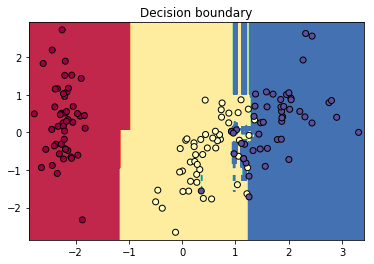Clearly for a nice dataset like Iris, ensemble learning is not needed. A simple 2-split decision tree gets the job done very well after principal component analysis.